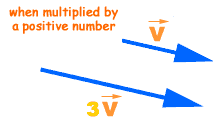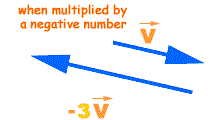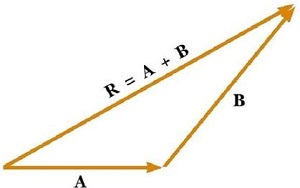# Topic: Motion in A Plane (Test 1)

Topic: Motion in A Plane
Q.1
The basic difference between a scalar and vector is one of
A. origin
B. direction
C. magnitude
D. polar angle
Explaination / Solution:

Scalar quantity gives you an idea about how much of an object there is, but vector quantity gives you an indication of how much of an object there is and that also in which direction. So, the main difference between these two quantities is associated with the direction, i.e. scalars do not have direction but vectors do.

Workspace
Report
Q.2
B. adding the angles of the vectors
C. translating the two vectors
D. adding the magnitudes of the vectors
Explaination / Solution:

The sum of two or more vectors is called the resultant. The resultant of two vectors can be found using either the triangle method or the parallelogram method.

Workspace
Report
Q.3
Magnitude of displacement of a particle is
A. is more than the path length of the particle between two points
B. is less than the path length of the particle between two points
C. is either less or equal to the path length of the particle between two points
D. is equal to the path length of the particle between two points
Explaination / Solution:

The maximum possible value for displacement is the distance travelled, so it cannot be of a greater value than distance (path length). The magnitude of the displacement is always less than or equal to the distance traveled. If two displacements in the same direction are added, then the magnitude of their sum will be equal to the distance traveled.

Workspace
Report
Q.4
Two vectors are equal if
A. the magnitude is the same for both
B. the two vectors have opposite directions
C. the magnitude and direction are the same for both
D. the direction is the same for both
Explaination / Solution:

Equal vectors are vectors that have the same magnitude and the same direction. Equal vectors may start at different positions.

Workspace
Report
Q.5

Multiplying a vector    by a positive real number

A. gives a scalar that is  times the magnitude of
B. gives a vector  =     in a direction opposite to
C. gives a scalar that is  times the polar angle of
D. gives a vector  =    in the same direction as
Explaination / Solution:

When a vector is multiplied by a positive number (for example 2, 3 ,5, 60 unit etc.) or a scalar only its magnitude is changed but its direction remains the same as that of the original vector.Workspace
Report
Q.6

Multiplying a vector   by a negative real number

A. gives a scalar that is  times the magnitude of
B. gives a vector  in the same direction as
C. gives a vector  =  in a direction opposite to
D. gives a scalar that is  times the polar angle of
Explaination / Solution:

If a vector is multiplied by a negative number (for example -2, -3 ,-5, -60 unit etc.) or a scalar not only its magnitude is changed but its direction also reversed.Workspace
Report
Q.7
To find the sum of vectors A and B, we place vector B
A. so that its tail is at the head of the vector A
B. so that its tail is at the tail of the vector A
C. so that its direction is the same as that of vector A
D. so that its head is at the head of the vector A
Explaination / Solution:

Triangle law of vector addition states that when two vectors are represented by two sides of a triangle in magnitude and direction taken in same order then third side of that triangle represents in magnitude and direction the resultant of the vectors.Taken in same order mean tail of Vector B must coincide with head of vector A as shown in image.

Workspace
Report
Q.8
A. intransitive
B. commutative
C. asymmetric
D. non-commutative
Explaination / Solution:

Vector addition is commutative, just like addition of real numbers. If you start from a point say P,  you end up at the same spot no matter which displacement (a or b) you take first. The head-to-tail rule yields vector c for both a + b and b + a.

Workspace
Report
Q.9
The addition of vectors and the multiplication of a vector by a scalar together gives rise to
A. intransitive law
B. distributive laws
C. asymmetric laws
D. commutative law
Explaination / Solution:

If a vector is multiplied by a scalar as in $p\stackrel{}{A}$ ,  then the magnitude of the resulting vector is equal to the product of p and the magnitude of ,   and its direction is the same as $\stackrel{}{A}$ if p is positive and opposite to $\stackrel{}{A}$ if p is negative.

Distributive law for scalar multiplication:

Workspace
Report
Q.10
Null vector or a zero vector has a magnitude
A. less than zero
B. equal to zero
C. that is complex
D. greater than zero